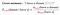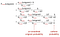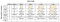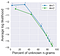# N-gram language models

## Part 2: Higher n-gram models

• To see the code I wrote for this project, you can check out its Github repo
• For other parts of the project: part 1, part 2, part 3

# Background

In part 1 of my project, I built a unigram language model: it estimates the probability of each word in a text simply based on the fraction of times the word appears in that text.

The text used to train the unigram model is the book “A Game of Thrones” by George R. R. Martin (called train). The texts on which the model is evaluated are “A Clash of Kings” by the same author (called dev1), and “Gone with the Wind” — a book from a completely different author, genre, and time (called dev2).

In this part of the project, I will build higher n-gram models, from bigram (n=2) all the way to 5-gram (n=5). These models are different from the unigram model in part 1, as the context of earlier words is taken into account when estimating the probability of a word.

# Higher n-gram language models

## Training the model

For a given n-gram model:

• The probability of each word depends on the n-1 words before it. For a trigram model (n = 3), for example, each word’s probability depends on the 2 words immediately before it.
• This probability is estimated as the fraction of times this n-gram appears among all the previous (n-1)-grams in the training set. In other words, training the n-gram model is nothing but calculating these conditional probabilities from the training text.

The example below shows the how to calculate the probability of a word in a trigram model:For simplicity, all words are lower-cased in the language model, and punctuations are ignored. The presence of the [END] tokens are explained in part 1.

## Dealing with words near the start of sentence

In higher n-gram language models, the words near the start of each sentence will not have a long enough context to apply the formula above. To make the formula consistent for those cases, we will pad these n-grams with sentence-starting symbols [S]. Below are two such examples under the trigram model:

From the above formulas, we see that the n-grams containing the starting symbols are just like any other n-gram. The only difference is that we count them only when they are at the start of a sentence. Lastly, the count of n-grams containing only [S] symbols is naturally the number of sentences in our training text:

## Dealing with unknown n-grams

Similar to the unigram model, the higher n-gram models will encounter n-grams in the evaluation text that never appeared in the training text. This can be solved by adding pseudo-counts to the n-grams in the numerator and/or denominator of the probability formula a.k.a. Laplace smoothing. However, as outlined part 1 of the project, Laplace smoothing is nothing but interpolating the n-gram model with a uniform model, the latter model assigns all n-grams the same probability:Laplace smoothing for unigram model: each unigram is added a pseudo-count of k. N: total number of words in training text. V: number of unique unigrams in training text.

Hence, for simplicity, for an n-gram that appears in the evaluation text but not the training text, we just assign zero probability to that n-gram. Later, we will smooth it with the uniform probability.

## Evaluating the model

Once all the conditional probabilities of each n-gram is calculated from the training text, we will assign them to every word in an evaluation text. Furthermore, the probability of the entire evaluation text is nothing but the products of all n-gram probabilities:

As a result, we can again use the average log likelihood as the evaluation metric for the n-gram model. The better our n-gram model is, the probability that it assigns to each word in the evaluation text will be higher on average.

# Coding the n-gram models

## Training the model

1. We build a `NgramCounter` class that takes in a tokenized text file and stores the counts of all n-grams in the that text. This class is almost the same as the `UnigramCounter` class for the unigram model in part 1, with only 2 additional features:

• For each sentence, we count all n-grams from that sentence, not just unigrams. Thankfully, the `nltk.util.ngrams` function can generates n-grams of any length from a list of sentence tokens:
• For each generated n-gram, we increment its count in the `counts` attribute of the class, which is a dict that maps each n-gram to their overall count in the training text. In additional, if that n-gram is at the start of the sentence — such as the bigram ‘i have’ — we also need to increment its sentence-starting count in additional to its overall count. This type of count will be stored in the `start_counts` attribute of the class.

For example, below is count of the trigram ‘he was a’. It appears 39 times in the training text, including 24 times at the beginning of a sentence:

2. The `NgramModel` class will take as its input an `NgramCounter` object. When the `train` method of the class is called, a conditional probability is calculated for each n-gram: the number of times the n-gram appears in the training text divided by the number of times the previous (n-1)-gram appears.

• These counts are taken from the `counts` attribute of the counter. An exception is the unigram model, in which there is no previous (n-1)-gram. Instead, we just divide the number of times the unigram appears in the training text by the total number of tokens in the text (stored in the counter’s `token_count` attribute).
• The resulting probability is stored in the `probs` attribute of the model, which is a dict that maps each n-gram to its overall conditional probability in the training text. Below is a example for the ‘he was a’ trigram:

However, if this n-gram appears at the start of any sentence in the training text, we also need to calculate its starting conditional probability:

• In this case, the counts of the n-gram and its corresponding (n-1)-gram are found in the `start_counts` attribute of the `NgramCounter`. Since unigrams do not have a corresponding (n-1)-gram, the starting probability of each unigram is the number of times it appears at the start of a sentence divided by the number of sentences in the training text (stored in the counter’s `sentence_count` attribute).
• The resulting probability is stored in the `start_probs` attribute of the model, which is a dict that maps each n-gram to its starting conditional probability in the training text. Note that the starting probabilities for the 4-gram and 5-gram models are the same in the example above. As a result, they are calculated only once and stored as a single key in the `start_probs` dict.

## Evaluating the model

Once all the n-gram conditional probabilities are calculated from the training text, we can use them to assign probability to every word in the evaluation text. Here, we take a different approach from the unigram model: instead of calculating the log-likelihood of the text at the n-gram level — multiplying the count of each unique n-gram in the evaluation text by its log probability in the training text — we will do it at the word level.

More specifically, for each word in a sentence, we will calculate the probability of that word under each n-gram model (as well as the uniform model), and store those probabilities as a row in the probability matrix of the evaluation text. As a result, this probability matrix will have:

• A width of 6: 1 uniform model + 5 n-gram models
• A length that equals the number of words in the evaluation text: 353110 for dev1, 450066 for dev2.V: number of unique unigrams in training text. N: total number of tokens in training text. Equal signs: identical starting probabilities, which are stored only once in the start_probs dict of the model

## Building the probability matrix

1. For the uniform model, we just use the same probability for each word i.e. 1/number of unique unigrams in training text. As a result, we can just set the first column of the probability matrix to this probability (stored in the `uniform_prob` attribute of the model).

`prob_matrix[:, 0] = self.uniform_prob`

2. To fill in the n-gram probabilities, we notice that the n-gram always end with the current word in the sentence, hence:

`ngram_end = token_position + 1`

• For a given n-gram, the start of the n-gram is naturally the end position minus the n-gram length, hence:

`ngram_start = token_position + 1 — ngram_length`

• If this start position is negative, that means the word appears too early in a sentence to have enough context for the n-gram model. An example would be the word ‘have’ in the above example: its `token_position` is 1, and its `ngram_length` is 3 under the trigram model. As a result, its `ngram_end` is 1+1=2, and its `ngram_start` is 2–3=-1. This makes sense, since the longest n-gram that it can make with the previous words is only the bigram ‘i have’. In other words, the trigram that ends in ‘have’ must be padded with a starting symbol [S]:
• In that case, the conditional probability simply becomes the starting conditional probability : the trigram ‘[S] i have’ becomes the starting n-gram ‘i have’. We get this probability by resetting the start position to 0 — the start of the sentence — and extract the n-gram until the current word’s position. We then obtain its probability from the `train_probs` attribute of the model. If the ngram is not found in this dict i.e. the n-gram did not appear in the training text, we give it a probability of zero as outlined earlier.
`if ngram_start < 0:    ngram = tuple(sentence[0:ngram_end])    prob_matrix[row, ngram_length] = self.start_probs.get(ngram, 0)`
• Otherwise, if the start position is greater or equal to zero, that means the n-gram is fully contained in the sentence, and can be extracted simply by its start and end position. We then retrieve its conditional probability from the `probs` attribute of the trained model. If the n-gram is not found in this dict, its probability is also set to zero.
`else:    ngram = tuple(sentence[ngram_start:ngram_end])    prob_matrix[row, ngram_length] = self.probs.get(ngram, 0)`

All of the above procedure are done within the `evaluate` method of the `NgramModel` class, which takes as input the file location of the tokenized evaluation text. It then reads each word in the tokenized text, and fills in the corresponding row of the that word in the probability matrix. Below is the code to train the n-gram models on train and evaluate them on dev1. The top 3 rows of the probability matrix from evaluating the models on dev1 are shown at the end.

## Model interpolation

Storing the model result as a giant matrix might seem inefficient, but this makes model interpolations extremely easy: an interpolation between a uniform model and a bigram model, for example, is simply the weighted sum of the columns of index 0 and 2 in the probability matrix.

The average log likelihood of the evaluation text can then be found by taking the log of the weighted column and averaging its elements. Below is one such example for interpolating the uniform model (column index 0) and the bigram model (column index 2), with weights of 0.1 and 0.9 respectively — note that models weight should add up to 1:

In the above example, dev1 has an average log likelihood of -9.36 under the interpolated uniform-bigram model. This interpolation method will also allow us to easily interpolate more than two models and implement the expectation-maximization algorithm in part 3 of the project.

# Result

We evaluate the n-gram models across 3 configurations:

1. N-gram length: from unigram (n=1) all the way to 5-gram (n=5)
2. N-gram interpolation weight: For each n-gram model, we interpolate it with the uniform model at different mixture weight: from 0 (0% n-gram, 100% uniform), to 0.3, 0.6, and 0.9 (90% n-gram, 10% uniform). Note that the n-gram model can never be 100%, since the n-grams with zero probability will drag the average log likelihood toward negative infinity.
3. Evaluation text: We evaluate the model on the training text itself (train), and also on the two evaluation texts (dev1 and dev2).

## Result

The graph below shows the average likelihoods across n-gram models, interpolation weights, and evaluation text.

There are quite a lot to unpack from the above graph, so let’s go through it one panel at a time, from left to right.

## Left panel: train

• As the n-gram increases in length, the better the n-gram model is on the training text. This is natural, since the longer the n-gram, the fewer n-grams there are that share the same context. As a result, this n-gram can occupy a larger share of the (conditional) probability pie.
• This phenomenon is illustrated in the below example of estimating the probability of the word ‘dark’ in the sentence ‘woods began to grow dark’ under different n-gram models:

From the above example of the word ‘dark’, we see that while there are many bigrams with the same context of ‘grow’ — ‘grow tired’, ‘grow up’ — there are much fewer 4-grams with the same context of ‘began to grow’ — the only other 4-gram is ‘began to grow afraid’. As a result, ‘dark’ has much higher probability in the latter model than in the former.

## Middle panel: dev1

• As we move from the unigram to the bigram model, the average log likelihood of dev1 slightly increases. This is likely due to the common bigrams that the two books share, since they are from the same series of the same author.
• In particular, the cases where the bigram probability estimate has the largest improvement compared to unigram are mostly character names. For example, given the unigram ‘lorch’, it is very hard to give it a high probability out of all possible unigrams that can occur. However, if we know the previous word is ‘amory’, then we are certain that the next word is ‘lorch’, since the two words always go together as a bigram in the training text.
• However, as we move from bigram to higher n-gram models, the average log likelihood drops dramatically! This bizarre behavior is largely due to the high number of unknown n-grams that appear in dev1 but not in train. They will have a probability of zero, which significantly drags down the average log likelihood of the evaluation text. Below is the breakdown of the number of unknown n-grams for each model, as well the percentage of words in the evaluation text that are unknown:

The above behavior highlights a fundamental machine learning principle:

A more complex model is not necessarily better, especially when the training data is small.

In our case, small training data means there will be many n-grams that do not appear in the training text. This problem is exacerbated when a more complex model is used: a 5-gram in the training text is much less likely to be repeated in a different text than a bigram does.

## Right panel: dev2

When the same n-gram models are evaluated on dev2, we see that the performance in dev2 is generally lower than that of dev1, regardless of the n-gram model or how much it is interpolated with the uniform model. This can be attributed to 2 factors:

1. Difference in n-gram distributions: from part 1, we know that for the model to perform well, the n-gram distribution of the training text and the evaluation text must be similar to each other. In this regard, it makes sense that dev2 performs worse than dev1, as exemplified in the below distributions for bigrams starting with the word ‘the’:

From the above graph, we see that the probability distribution of bigram starting with ‘the’ is roughly similar between train and dev1, since both books share common definite nouns (such as ‘the king’). In contrast, the distribution of dev2 is very different from that of train: obviously, there is no ‘the king’ in “Gone with the Wind”.

2. Unknown n-grams: since train and dev2 are two books from very different times, genres, and authors, we should expect dev2 to contain many n-grams that do not appear in train. In fact, if we plot the average log likelihood of the evaluation text against the fraction of these “unknown” n-gram (in both dev1 and dev2), we see that:Number above each data point indicates the n-gram length of the model
• There is a strong negative correlation between fraction of unknown n-grams and average log likelihood, especially for higher n-gram models such as trigram, 4-gram, and 5-gram.
• For a given n-gram model, dev2 always has higher fraction of unknown n-gram than dev1. It is no surprise, then, that dev2 generally has a lower average log likelihood than dev1.

## Effect of model interpolation

A common thread across these observations is that regardless of the evaluation text (dev1 and dev2), and regardless of the n-gram model (from unigram to 5-gram), interpolating the model with a little bit of the uniform model generally improves the average log likelihood of the model.

The effect of this interpolation is outlined in more detail in part 1, namely:

1. Interpolating with the uniform model gives a small probability to the unknown n-grams, and prevents the model from completely imploding from having n-grams with zero probabilities. This explains why interpolation is especially useful for higher n-gram models (trigram, 4-gram, 5-gram): these models encounter a lot of unknown n-grams that do not appear in our training text.

2. Interpolating with the uniform model reduces model over-fit on the training text. Of course, the model performance on the training text itself will suffer, as clearly seen in the graph for train. However, the model can generalize better to new texts that it is evaluated on, as seen in the graphs for dev1 and dev2.

# Final remarks

This part of the project highlights an important machine learning principle that still applies in natural language processing: a more complex model can be much worse when the training data is small!

For n-gram models, this problem is also called the sparsity problem, since no matter how large the training text is, the n-grams within it can never cover the seemingly infinite variations of n-grams in the English language. In other words, many n-grams will be “unknown” to the model, and the problem becomes worse the longer the n-gram is.

In the next part of the project, I will try to improve on these n-gram model. For example, instead of interpolating each n-gram model with the uniform model, we can combine all n-gram models together (along with the uniform). We can further optimize the combination weights of these models using the expectation-maximization algorithm.

## References

Chapter 3 of Jurafsky & Martin’s “Speech and Language Processing” is still a must-read to learn about n-gram models. Most of my implementations of the n-gram models are based on the examples that the authors provide in that chapter.

Written by

Written by

## Khanh Nguyen

#### Data scientist based in Ho Chi Minh City, Vietnam | dknguyen.com